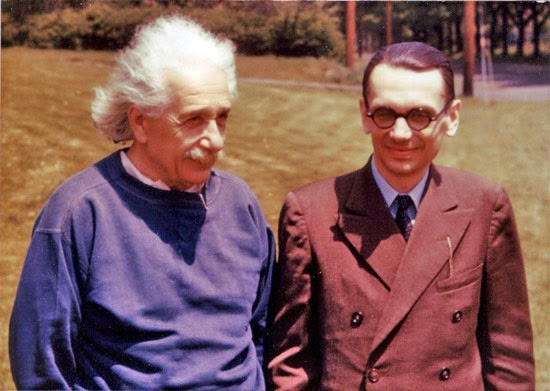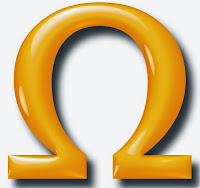## Saturday, May 3, 2014

### The Divine Random in Mathematics

I am the rock, you the stone,
Together we ballast reality.
Whither the sheets above us are blown.
Until fairly recently, 1931 to be exact, scientists and mathematicians believed we would someday find a theory that explains everything. By "theory" I mean a Formal Axiomatic System (FAS). A FAS is simply a relatively small set of given facts, axioms, from which a much larger set of theorems can be proven "by mechanical means." The axioms in a FAS are so basic that we believe them to be true without the need for further justification. A famous and straightforward example of a FAS is the small set of axioms provided by Giuseppe Peano at the end of the 19th century to formally define the natural numbers. The Peano axioms, in English, are as follows:
1. The natural numbers contain the number 1.
2. Every natural number has a successor, the next natural number.
3. The number 1 is not the successor of any natural number.
4. Two different natural numbers cannot have the same successor.
5. If a set contains the successors of each of its members and the number 1, then it contains all the natural numbers.

From this small set of seemingly obvious "facts" and using the mechanical rules of logic, all of number theory can be derived. Addition, multiplication, prime numbers, prime decomposition and the Fundamental Theorem of Arithmetic, are all consequences of the five Peano Axioms listed above. Or, to look at it the other way round, the Peano Axioms constitute a very efficient compression of the entire body of number theory.

And that is how mathematics works. Every branch of it — geometry, algebra, analysis, etc. — can be boiled down to a similar compression of a very large body of theorems into a relatively small set of axioms. Unlike other sciences such as physics, biology, chemistry, or computation, mathematics is unconstrained by the laws of the universe in which we find ourselves living. It is purely an invention of man, limited only by our evolving intellect. We invented the game and control the rules. Surely, there are no fundamental reasons why we couldn't develop a mathematical Theory of Everything (ToE), a FAS from whose axioms one can derive by mechanical means all that is true.Albert Einstein and Kurt Gödel
That is what pretty much every mathematician believed until 1931 when a 25 year-old Austrian post-doc named Kurt Gödel shocked everyone by proving that mathematics, that wholly man-made "queen of sciences," suffers from the same kind of fundamental limitation Heisenberg had discovered in physics five years earlier and Turing would find for computation five years later. Gödel's meta-theorem proved there is a shoreline beyond which vast shoals of truth exist that the lighthouse of pure mathematics cannot illuminate.

More specifically (and much less metaphorically), Gödel showed that for any FAS that is at least as rich as the Peano Axioms described above, and for which the axioms are not self-contradictory, there will be theorems expressible in the language of the FAS that are true, but un-provable from the axioms. That is, any consistent FAS is necessarily incomplete. Pure mathematics is not sufficient to account for a ToE. Truth, with a capital T, is incompressible. There will always be a theorem, perhaps infinitely many, whose shortest expression is the theorem itself. The only way to prove such theorems is to add them to the set of axioms!

Like other impossibility meta-theorems, e.g., Heisenberg's Uncertainty Principle in physics and Turing's Undecidability result in computing, Gödel's Incompleteness Theorem identifies a fundamental limit on our ability to know things, in this case using the tools of mathematics. As we have seen for physics and computing, beyond these boundaries lies randomness, and the same appears to be true for math as well. Our simple working definition of randomness, that it be universally unpredictable, can be made more precise in the realm of mathematics. Namely, something is mathematically random if there exists no FAS (with a finite set of axioms) from which it can be derived. A random number, in particular, is a number whose digits cannot be predicted within the framework of mathematics. Such a number is irreducible — the shortest possible way to express one is to write down all its (infinitely many) digits. If we can agree that a name for something is just a finite sequence of symbols that represent it, these numbers are necessarily nameless. Even numbers like π and e, which are transcendental, their digits never repeat, have namesπ and e are the names we have given to two specific infinite real numbers that can be computed in two very specific, and finite, ways. Mathematically random (aka irreducible) numbers are even weirder than transcendentals. So weird that it becomes reasonable to ask, do such numbers actually exist, and if so, can you show me one?

It's actually pretty easy to see that irreducible numbers, numbers that cannot be derived within any FAS, do indeed exist. However complex pure mathematics may become, it must eventually have a finite set of axioms from which all theorems, including those showing the existence of certain numbers, can be mechanically proved. A finite set of axioms can be used to derive an infinite set of theorems, just like a finite alphabet can generate an infinite set of names. But in both cases, the infinity of derivations is a countable infinity — there are only as many of them as there are integers. On the other hand, there is an uncountably infinite number of real numbers, which is a bigger infinity. So if you match up numbers to name/theorems, you will always have more of the former than the latter. In other words, there must be an (uncountably) infinite number of irreducible numbers. This is a so-called diagonalization argument that can be made formally rigorous, but is never very satisfying. What we'd really like to do is hold one of these odd beasts in our hands and examine it up close.

One obvious way to generate such a number is to appeal back to physics. We could simply flip a fair coin and write 0 for heads or 1 for tails, and continue doing that to generate as many bits as desired. Assuming we trust the fairness of our fair coin, the bits (digits) of such a number would be universally unpredictable — every bit is a completely separate "fact" and there is no shorter description of how to obtain them other than the number itself. But is there a way to do this while staying within the confines of pure math?Around the time The Beatles brought bangs to America, in 1966, Dr. Gregory Chaitin found an answer to this question when he discovered what he called the Omega Numbers. Each Ω (there are infinitely many) is definable but completely irreducible. Like π and e, Ω is a transcendental number, but unlike it's more well-behaved cousins, Ω cannot be computed by any algorithm. The smallest possible expression of its digits are the digits themselves. Furthermore, those digits are normally distributed — every digit appears approximately the same number of times — no matter what base (decimal, octal, binary, hexadecimal, etc.) one might choose to represent it. Ω is mathematically random. To me, the most fascinating thing about Ω is that it sits at the nexus of Gödel's incompleteness and Turing's undecidability, telling us that the oceans of randomness beyond mathematics and computing are, in fact, one and the same — the Divine Random.

Chaitin defines Ω using the terminology and formalism of Turing (which, if you'll recall, is still within the realm of pure mathematics) by first choosing a suitable formal programming language and initializing an estimate of Ω to zero. For Chaitin's purposes, this means the language must be prefix-free so that programs are self-delimited. We then enumerate every possible string in the language. For each string we ask, a) is the string a syntactically correct program with input data appended, and b) does that program halt when supplied with the input data? If the answers to both a) and b) are Yes, we add the following quantity to our estimate: 1/2|p|, where |p| is the number of bits required to represent the program in the chosen language. If not, we just continue combing through all possible strings looking for legal, halting programs.

Does such a procedure produce a well-defined number? Absolutely! It is surely possible to lexically enumerate all possible strings, every string is either a legal program+data or not, and every legal program+data either halts or it doesn't. In fact, Chaitin showed that the resulting number, Ω, is a real number between 0 and 1. The catch, of course, is that it is impossible to compute such a number because, as Turing proved, it isn't possible to compute the answer to the halting problem in full generality. In a certain sense, each bit of Ω is chosen by flipping a mathematical coin — heads the program halts, tails it doesn't — whose outcome is universally unpredictable and uncomputable.

So what does it look like, you must be asking? Well, I can't say. As with other objects we have encountered floating in the sea of randomness, Ω can be seen only by its faint, hazy outline. We can begin to estimate Ω but we can never know more than a finite number of its digits. Moreover, it is impossible to compute how accurate our estimate is, how close or far away it is to the actual number. Ω will always remain fuzzy, its properties uncertain beyond some degree of magnification, much like the properties of physical objects governed by Heisenberg's Uncertainty Principle. Chaitin put it best in his excellent and accessible little monograph Meta Math! The Quest for Omega:

So fixing randomness in your mind is like trying to stare at something without blinking and without moving your eyes. If you do that, the scene starts to disappear in pieces from your visual field.

The harder you stare at randomness, the less you see of it!
Even if we can't know exactly what it looks like, the very existence of Ω proves, or at least strongly suggests, Gödel's incompleteness meta-theorem. There are indeed theorems, and numbers, that cannot be compressed, that cannot be derived by any effective procedure from a smaller set of axioms. And we know that mathematics is limited in this way because Turing showed us there are limits to what any effective procedure, aka computer program, aka Finite Axiomatic System, can accomplish. No matter how clever we humans are or will become, there are barriers to our knowledge that cannot be scaled. Tantalizingly, the answers are out there, floating around as random numbers. Ω, for example, solves the halting problem for every possible program. And it exists; it's out there, we just aren't allowed to see it. Similarly, there exists a random number whose binary bits answer every conceivable yes/no question that can be posed in a given language, including questions like, "Is Goldbach's conjecture true?" Science can't give us all those answers. Physics, mathematics, and computation theory are well-honed tools, but they cannot carve all the universe.

We are now approaching the point at which you might begin to understand why I call it, The Divine Random.